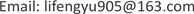1. 引言

σ 2 m = 1 + ε D v ρ p 2 ( 1 − 1 − e − α T α T ) = 1 + Y (1)

Y = ε D v ∑ i = 1 7 2 A i α i H 0 ( α i ) ( 1 − 1 − e − α i T α i T ) (2)

A i 、 α i 由下面的零功率传递函数决定，

H 0 ( s ) = ∑ i = 1 7 A i α i + s

2. 临界发散的问题

〈 ( c k + 1 − c k ) 2 〉 2 〈 c k 〉 = 1 + W (3)

〈 ⋅ 〉 表示均值， c k 为第k个时间间隔T内的计数，W反映了堆内中子相关程度。

Wallerbos  发现由于试验测量时间是有限的，实际试验中相关中子测度Y并不会发散，提出了修正后的有限测量时间模型

〈 Y ( N T ) 〉 = 2 ε D v ∑ i = 1 7 A i α i H 0 ( α i ) × [ f ( α i T ) − 1 N f ( α i N T ) ] (4)

NT为测量时间， f ( T ) = 1 − 1 − e − α T α T 。如果测量时间无限， N → ∞ ，则 Y ( T ) 的期望值

lim N → ∞ 〈 Y ( N T ) 〉 = 2 ε D v ∑ i = 1 7 A i α i H 0 ( α i ) f ( α i T )

lim x → 0 f ( x ) = 1 2 x − 1 6 x 2

lim α 7 → 0 A 7 α 7 H 0 ( α 7 ) [ f ( α 7 T ) − 1 N f ( α 7 N T ) ] = 1 12 A 7 2 ( N − 1 ) T 2

3. 计数时间间隔效应

c ¯ = ∑ i = 1 N c i ， c 2 ¯ = ∑ i = 1 N c i 2 ¯ + 2 ∑ i < j c i c j ¯

Y ( N T ) = 2 ε D v ∑ i = 1 7 A i α i H 0 ( α i ) [ 1 − 1 − e − α i T α i T + ( 1 − e − α i T ) 2 α i T     × ∑ j = 1 N N − j N exp ( − α i ( j Δ + j − 1 ) T ) ] (5)

4. 使用多个探测器的问题

Y s y n ( T ) = ( 1 ∑ i = 1 n ε i D ( d i ) ) { ∑ i = 1 n ε i 2 D 2 ( d i ) × [ Q T ( 2 − d i T ) ( − d i T ) + A ( 1 − d i T ) e − α d i f ( T − d i ) ]     + 2 ∑ i = 1 n ∑ j = i + 1 n ε i ε j D ( d i ) D ( d j ) A f ( T ) } (6)

n为探测器的个数， A = v ( v − 1 ) ¯ α 2 τ f 2 ， f ( T ) = 1 − 1 − e − α T α T ，Q为裂变率， τ f 为发生一次裂变的平均时间， D ( d i ) 是关于不灵敏时间 d i 的函数，其余符号含义同前。Yamane讨论了n个探测器的效率 ε i 和不灵敏时间 d i 都相同均为 ε 和d的情形，假设不灵敏时间d与测量时间T之比 d / T 很小， f ( T − d ) 几乎等于 f ( T ) ，则(6)简化为

Y s y n ( T ) = − 2 ε D ( d ) Q d + n ε D ( d ) A ( 1 − 1 − e − α T α T ) 不考虑不灵敏时间效应， d = 0 ， D ( d i ) = 1 ，进一步简化为

Y s y n ( T ) = n ε A ( 1 − 1 − e − α T α T ) (7)

5. 中子源释放相关中子

p ( t 1 , t 2 ) = p c o r r ( t 1 , t 2 ) + p ( t 1 ) p ( t 2 ) = p c , f ( t 1 , t 2 ) + p c , s ( t 1 , t 2 ) + p ( t 1 ) p (t2)

p c , f ( t 1 , t 2 ) 表示由同一裂变链引起中子相关的几率， p c , s ( t 1 , t 2 ) 表示由中子源引起中子相关的几率， p ( t 1 ) 、 p ( t 2 ) 分别是在 ( t 1 , t 1 + d t 1 ) 和 ( t 2 , t 2 + d t 2 ) 时间间隔内各自独立探测到一个中子的几率。在不考虑缓发中子效应时，Pazsit得到了此时计算相关中子测度Y的方法 

Y ( T ) = ε D v 1 ρ 2 ( 1 + δ ) ( 1 − 1 − e − α T α T ) (8)

6. 小结